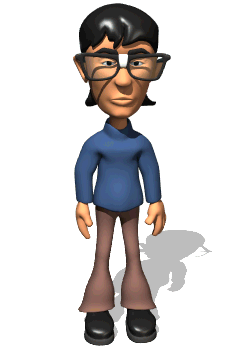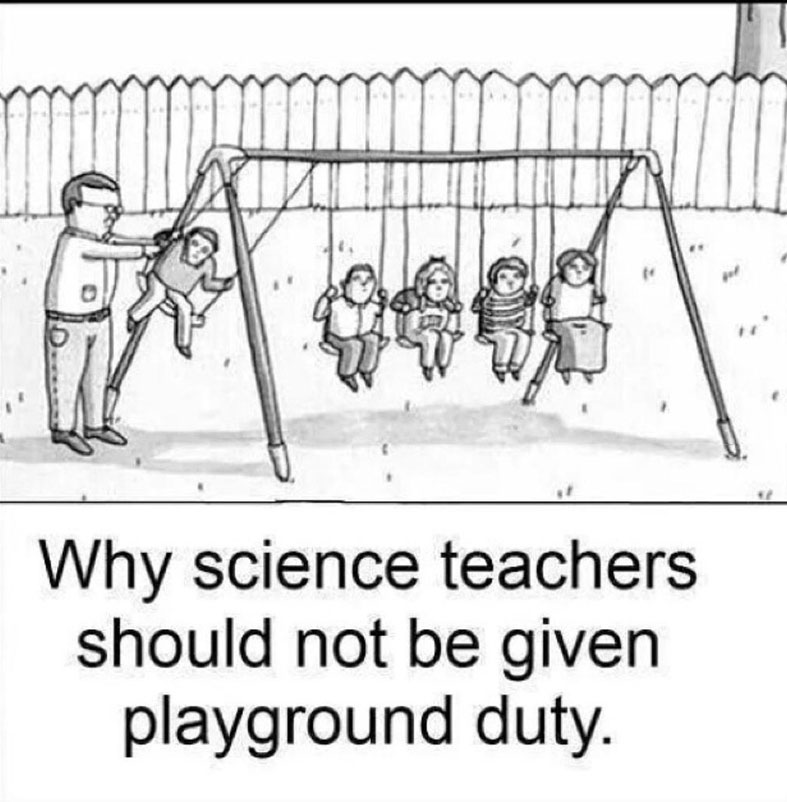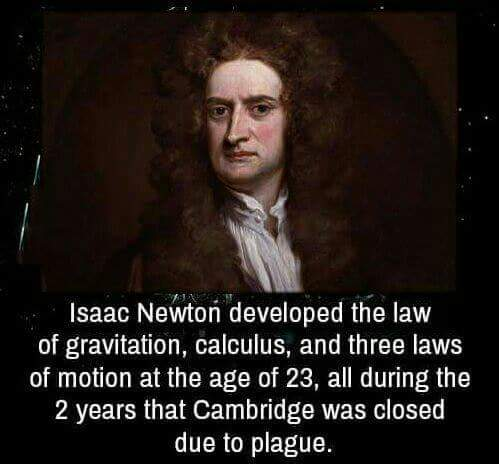Video Instruction

showmethephysics.com

Visualizing Newton's 3rd Law

- Frank Noschese - John Jay H.S.

Action/Reaction - NASA
(right click, save as)"Which way is the skater below moving?""All the forces in the universe, would  be: a) an even number b) odd c) unknowable

Even Number!!!

Why?

3rd Law of Motion

 For every action there is an equal but opposite reaction

"Press on a rock and the rock presses on you
(with an equal, but opposite force)"

- Newton

[ Flash ]Skateboarder

[ HTML5 ]When object A exerts  a force on object B, Object B exerts an equal force on A but in the opposite direction

Forces Always
Come in Pairs[ HTML5 ]
Show Me The PhysicsA lamp applies a force of 10 N to a table.

What force does the table
apply on the lamp?

Which direction is this force applied?

[ Flash ]

UPEarth pulls
down on you.

That's half the story...

You pull up on the earth.

Equal.

Opposite.

Explain this phenomena using one or more
of Newton's Laws of Motion

Magnetically Coupled Pendula 2

School Blocks YouTube? Use the file below.

The more active bouncing magnet applies a force on the slower magnet on the opposite side. By applying a force it receives a force and therefore slows down.Paul Hewitt Question

A heavy rock and a light rock in free fall (zero air resistance) have the same acceleration. The reason the heavy rock doesn’t have a greater acceleration is that the

A. force due to gravity is the same on each.

B. air resistance is always zero in free fall.

C. inertia of both rocks is the same.

D. ratio of force to mass is the same.

E. None of these.

Isaac NewtonFree Body DiagramsRotational Inertia